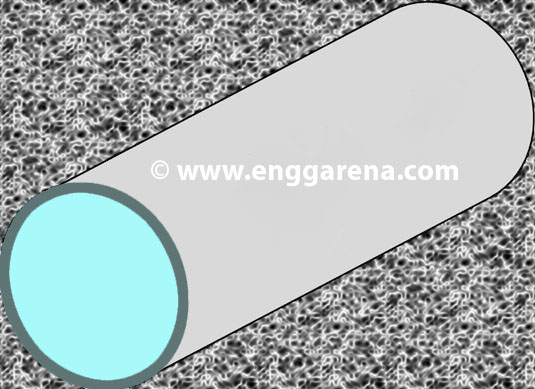Feb 26, 2016

Feb 26, 2016

Feb 26, 2016

# Headrace Pressure Flow in Steel PipesHeadrace pipe can also serve the same function as that of Headrace tunnels, depending upon the site location, geological and topographical constraints. as discussed earlier that tunnels can be divided into two types on the basis of type of flow

• Pressure Flow Tunnels
• Gravity Flow Tunnels.

Similarly headrace pipes can also be divided into two categories pressure flow and gravity flow. Following are the design examples of the two types:

## Hydraulic Design of Pressure Flow in Headrace Pipes (STEEL)

Let suppose a 50 cumecs needs to be passed through 4.0m diameter steel pipe with a total length of 1000m, longitudinal slope of 1/1000 m, Mannng’s coefficient of 0.014 (steel) and with a gross head of 100m.

### BASIC DATA

Pipe invert elevation at Start

I.L.1

=

100.00

m a.s.l.

Design discharge

Q

=

50.0

m3/s

Internal diameter of pipe

D

=

4.00

m

Flow velocity

V

=

4.0

m/s

R

=

1.00

m

H

=

100.00

m

Effective head from center of pipe

he

=

10.0

m

Max. possible flow velocity (orifice flow)

VOmax

=

8.40

m/s

Max. possible discharge (orifice flow)

QOmax

=

105.61

m3/s

Alternatively Using another equation

Max. possible discharge (conduit flow)

QCmax

=

147.1

m3/s

Check QOmax ≥ Q

=

O.K.

Total length of pipe (Δx)

L

=

1000.0

m

Longitudinal slope

S

=

0.0010

m/m

Hazen-Williams roughness coefficient

C

=

120

Mannings friction factor (steel)

n

=

0.0140

Friction factor (Moody)

f

=

0.0130

FRICTION FACTOR

Using Reynolds number

Kinematic viscosity of water at 20oC

ν

=

1.0E-06

m2/s

Reynolds number

Re

=

15915494

Check Re ≤ 2000

=

TURBULENT

Roughness height (welded steel)

e

=

0.600

mm

Ratio

e/D

=

0.00015

1

=

1.00

Friction factor (Moody)

f

=

0.01304

SUBMERGENCE REQUIREMENTS

Minimum submergence (by Knauss)

Smin

=

9.84

m

" " " " (by Rohan)

Smin

=

8.20

m

" " " " (by Gordon)

Smin

=

5.77

m

Minimum submergence

Smin

=

7.94

m

Provided submergence

SPr

=

10.00

m

Check SPr ≥ Smin

=

O.K.

FRICTION LOSS

hf

=

3.10

m

hf

=

2.46

m

hf

=

2.63

m

Using Colebrook - White equation

1

=

1.00

Friction loss (Colebrook-White)

hf

=

2.63

m

hfmax

=

3.10

m

BEND LOSS

No. of bends

nB

=

10

no.

hLB

=

1.21

m

ENTRANCE LOSS

Velocity at pipe bellmouth

vE

=

0.46

m/s

hLE

=

0.0011

m

hLT

=

4.31

m

WALL THICKNESS

Bulk modulus of water

k

=

2.1E+09

N/m2

Modulus of elasticity of pipe material

E

=

2.1E+11

N/m2

Wall thickness (provided)

ts

=

25.0

mm

Hydrostatic pressure

Pw

=

10.00

kN/mm2

Weld efficiency

kf

=

0.95

Allowable tensile stress

σf

=

1400

kN/mm2

Corrosion allowance

cs

=

1.5

mm

Wall thickness (required)

ts

=

15.04

mm

Wall thickness (provided)

ts

=

25.0

mm

Density of steel

ρs

=

7850.0

kg/m3

Minimum thickness of steel sheet

tmin

=

11.25

mm

Effective thickness of pipe

te

=

23.50

mm

Check te ≥ tmin

=

O.K.

Hoop stress

σH

=

851.06

kgf/cm2

Check σH ≤ 1.5yield stress

=

O.K.

STEEL QUANTITY

X-sectional area of steel

As

=

0.316

m2

Volume of steel

Vs

=

316.12

m3

Weight of steel

Ws

=

2481.56

tons

Weight of steel (5% increse for joints)

Ws

=

2605.64

tons

Width of steel sheet

w

=

12.72

m

Length of steel sheet

l

=

6.0

m

No. of steel sheets

n

=

167

no.